##### The Japanese Bonsai specialist
Direct order Contact Help / Services Newsletter# Stone lantern yukimi gata 185 cm wooden window

Yukimi gata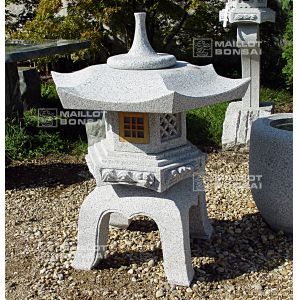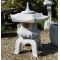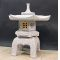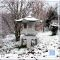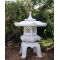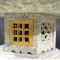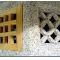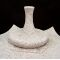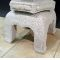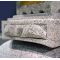ref. : 7420

1.640,00

voluminous and/or heavy product must be collected at nursery

Available quantity : 1Order

###### Description

Height 185 cm. Ø 150 cm, weight 1200 kilograms, delivered in 5 elements to be easily assembled at home. A free-standing lantern made of real granite with elegantly curved lines. It features a wooden window, stone latticework and a temple-style pointed roof. An electric cable can be passed through the centre to light a small lamp inside.

#lantern 5.7 #stone 5.5 #wooden 3.6 #window 3.6 #yukimi 2.6 #gata 2.4 #latticework 2.1 #elegantly 1.9 #kilograms 1.9 #delivered 1.9

Formule
(( ROUND((CHAR_LENGTH(b.article_nom)-CHAR_LENGTH(REPLACE(b.article_nom, 'lantern', '')))/LENGTH('lantern')) + ROUND((CHAR_LENGTH(b.article_description)-CHAR_LENGTH(REPLACE(b.article_description, 'lantern', '')))/LENGTH('lantern')) ) * 3.7) + (( ROUND((CHAR_LENGTH(b.article_nom)-CHAR_LENGTH(REPLACE(b.article_nom, 'wooden', '')))/LENGTH('wooden')) + ROUND((CHAR_LENGTH(b.article_description)-CHAR_LENGTH(REPLACE(b.article_description, 'wooden', '')))/LENGTH('wooden')) ) * 3.6) + (( ROUND((CHAR_LENGTH(b.article_nom)-CHAR_LENGTH(REPLACE(b.article_nom, 'window', '')))/LENGTH('window')) + ROUND((CHAR_LENGTH(b.article_description)-CHAR_LENGTH(REPLACE(b.article_description, 'window', '')))/LENGTH('window')) ) * 3.6) + (( ROUND((CHAR_LENGTH(b.article_nom)-CHAR_LENGTH(REPLACE(b.article_nom, 'stone', '')))/LENGTH('stone')) + ROUND((CHAR_LENGTH(b.article_description)-CHAR_LENGTH(REPLACE(b.article_description, 'stone', '')))/LENGTH('stone')) ) * 3.5) + (( ROUND((CHAR_LENGTH(b.article_nom)-CHAR_LENGTH(REPLACE(b.article_nom, 'yukimi', '')))/LENGTH('yukimi')) + ROUND((CHAR_LENGTH(b.article_description)-CHAR_LENGTH(REPLACE(b.article_description, 'yukimi', '')))/LENGTH('yukimi')) ) * 2.6) + (( ROUND((CHAR_LENGTH(b.article_nom)-CHAR_LENGTH(REPLACE(b.article_nom, 'gata', '')))/LENGTH('gata')) + ROUND((CHAR_LENGTH(b.article_description)-CHAR_LENGTH(REPLACE(b.article_description, 'gata', '')))/LENGTH('gata')) ) * 2.4) + (( ROUND((CHAR_LENGTH(b.article_nom)-CHAR_LENGTH(REPLACE(b.article_nom, 'latticework', '')))/LENGTH('latticework')) + ROUND((CHAR_LENGTH(b.article_description)-CHAR_LENGTH(REPLACE(b.article_description, 'latticework', '')))/LENGTH('latticework')) ) * 2.1) + (( ROUND((CHAR_LENGTH(b.article_nom)-CHAR_LENGTH(REPLACE(b.article_nom, 'elegantly', '')))/LENGTH('elegantly')) + ROUND((CHAR_LENGTH(b.article_description)-CHAR_LENGTH(REPLACE(b.article_description, 'elegantly', '')))/LENGTH('elegantly')) ) * 1.9) + (( ROUND((CHAR_LENGTH(b.article_nom)-CHAR_LENGTH(REPLACE(b.article_nom, 'kilograms', '')))/LENGTH('kilograms')) + ROUND((CHAR_LENGTH(b.article_description)-CHAR_LENGTH(REPLACE(b.article_description, 'kilograms', '')))/LENGTH('kilograms')) ) * 1.9) + (( ROUND((CHAR_LENGTH(b.article_nom)-CHAR_LENGTH(REPLACE(b.article_nom, 'delivered', '')))/LENGTH('delivered')) + ROUND((CHAR_LENGTH(b.article_description)-CHAR_LENGTH(REPLACE(b.article_description, 'delivered', '')))/LENGTH('delivered')) ) * 1.9)

## Secure payment## Delivery

Our logistic partners :